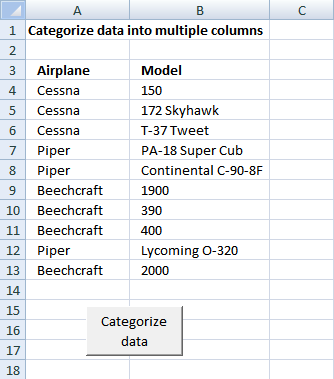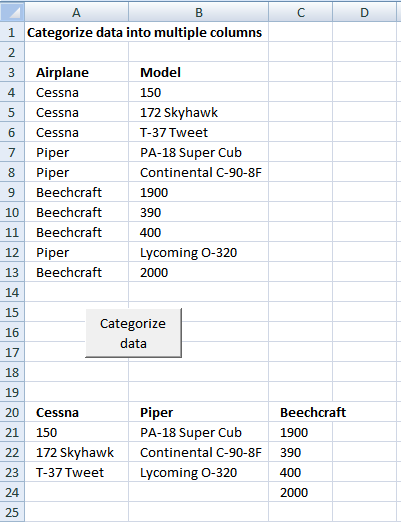Author: Oscar Cronquist Article last updated on December 18, 2018

In this post I am going to rearrange values from a list into unique columns.

Before:After:The code

```Sub Categorizedatatocolumns()

Dim rng As Range

Dim dest As Range

Dim vrb As Boolean

Dim i As Integer

Set rng = Sheets("Sheet1").Range("A4")

vrb = False

Do While rng <> ""

Set dest = Sheets("Sheet1").Range("A20")

Do While dest <> ""

If rng.Value = dest.Value Then

vrb = True

End If

Set dest = dest.Offset(0, 1)

Loop

If vrb = False Then

dest.Value = rng.Value

dest.Font.bold = True

End If

vrb = False

Set rng = rng.Offset(1, 0)

Loop

Set rng = Sheets("Sheet1").Range("A4")

Do While rng <> ""

Set dest = Sheets("Sheet1").Range("A20")

Do While dest <> ""

If rng.Value = dest.Value Then

i = 0

Do While dest <> ""

Set dest = dest.Offset(1, 0)

i = i + 1

Loop

Set rng = rng.Offset(0, 1)

dest.Value = rng.Value

Set rng = rng.Offset(0, -1)

Set dest = dest.Offset(-i, 0)

End If

Set dest = dest.Offset(0, 1)

Loop

Set rng = rng.Offset(1, 0)

Loop

End Sub
```

### Get excel tutorial file

Remember to backup your excel workbook, you can´t undo macros.
Categorize-data-into-multiple-columns.xls

(Excel 97-2003  Workbook *.xls)